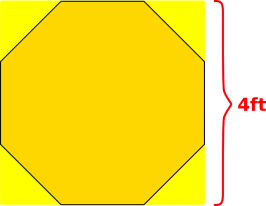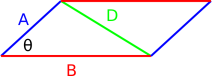[Contents]

## 1  Algebra / Trigonometry Quiz

Rationale: This math quiz is intended to test the skills you ought to have in preparation for an introductory physics class. It emphasizes word problems, and emphasizes the ability to think clearly and carefully. It requires you to know some algebra and trigonometry, but not assume you already know any calculus or physics.

Some of the questions that look hard may be easy, if you think about them the right way. Some of the problems that look easy may be slightly harder than they look.

Instructions: In all cases, show your work. Even if you can solve the problem in your head, briefly explain how you solved it. Closed book. No computers or electronic calculators; just pencil and paper.

1.    What is the remainder when 1,000,000,001 is divided by 9?

2.    As indicated in figure 1, we have two squares, partially overlapping. The side of one is equal to the diagonal of the other. We wish to compare the area of the red square to the area of the blue square. The ratio of areas is ________.Figure 1: Two Squares

3.    In general, the power dissipated in a resistor can be calculated using the formula P = I2 R. Consider a situation where the power is P = 50 watts and the resistance is R = 1 ohm. What is I? Please answer in numerical form, accurate to 1% or better.

4.    You are given a blank square piece of plywood, 4 ft on a side. The plan is to cut off the corners so as to make a regular octagon, which you can then paint to make a giant stop sign, as shown in figure 2. What is the length of each side of the resulting octagon? Also, what is the length of the legs of the triangles that get cut off? Please answer in numerical form, accurate to 1% or better.Figure 2: Octagon Inscribed in a Square

5.    We are given a cube 1 inch on a side. How long is the diagonal of the cube? An algebraic / symbolic answer suffices.

6.    Which is bigger, the sine of one degree, or the tangent of one degree?

7.    Find x such that x = √(6 + √(6 + √(6 + ⋯))) where “⋯” means “and so on, forever”.

8.    How many different ways are there of making change for a quarter? You may use pennies, nickels, and dimes. (When deciding what is a “different way” we do not care about the order in which the coins are dispensed, just how many coins of each type are used.)

Note for those who are unfamiliar with US currency: “quarter” = 25 cents; “dime” = 10 cents; “nickel” = 5 cents; “penny” = 1 cent.

9.    Let’s analyze a coin-tossing experiment. The coin is lopsided such that it comes up “heads” 60% of the time. Each toss is statistically independent of the others. We will toss the coin six times. What is the probability that it will come up “heads” exactly four times out of six?

10.    Given the parallelogram shown in figure 3, find a general expression for the length of the diagonal D in terms of the sides A and B and the angle θ.Figure 3: Generic Parallelogram

[Contents]